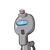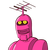# The cost of pencil box is Rs.15.70. Find the cost of 5 such pencilboxes​

The cost of pencil box is Rs.15.70. Find the cost of 5 such pencil
boxes​

### 2 thoughts on “The cost of pencil box is Rs.15.70. Find the cost of 5 such pencil<br />boxes​”

1.Cost of one pencil box =15.70

cost of 5 pencil box=cost of one pencil box ×5=. 15.70×5

₹ 78.5

Therefore ,the cost of 5 pencil box is ₹78.5

I hope it helps you

2.Rs.78.50

Step-by-step explanation:

The cost of 1 pencil box=Rs.15.70

The cost of 5 pencil boxes= Rs.15.70×5= Rs.78.50

So, 5 pencil boxes cost Rs.78.50

Hope it helps you...

THANK YOU!